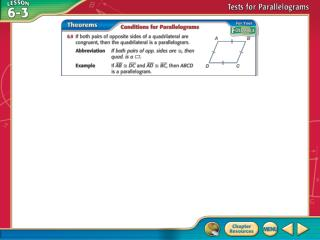Download PresentationConcept 1Concept 1 - PowerPoint PPT Presentation

Download PresentationConcept 1
An Image/Link below is provided (as is) to download presentation

Download Policy: Content on the Website is provided to you AS IS for your information and personal use and may not be sold / licensed / shared on other websites without getting consent from its author. While downloading, if for some reason you are not able to download a presentation, the publisher may have deleted the file from their server.

- - - - - - - - - - - - - - - - - - - - - - - - - - - E N D - - - - - - - - - - - - - - - - - - - - - - - - - - -
Presentation Transcript

1. Concept 1

2. Concept 2

3. Identify Parallelograms Determine whether the quadrilateral is a parallelogram. Justify your answer. Answer: Each pair of opposite sides has the same measure. Therefore, they are congruent.If both pairs of opposite sides of a quadrilateral are congruent, the quadrilateral is a parallelogram. Example 1

4. A B C D Which method would prove the quadrilateral is a parallelogram? A. Both pairs of opp. sides ||. B. Both pairs of opp. sides . C. Both pairs of opp. ’s . D. One pair of opp. sides both || and . Example 1

5. Use Parallelograms to Prove Relationships MECHANICS Scissor lifts, like the platform lift shown below, are commonly applied to tools intended to lift heavy items. In the diagram, A  C and B  D. Explain why the consecutive angles will always be supplementary, regardless of the height of the platform. Example 2

6. Use Parallelograms to Prove Relationships Answer: Since both pairs of opposite angles of quadrilateral ABCD are congruent, ABCD is a parallelogram by Theorem 6.10. Theorem 6.5 states that consecutive angles of parallelograms are supplementary. Therefore, mA + mB = 180 and mC + mD = 180. By substitution, mA + mD = 180 and mC + mB = 180. Example 2

7. A B C D The diagram shows a car jack used to raise a car from the ground. In the diagram, AD BC and AB  DC. Based on this information, which statement will be true, regardless of the height of the car jack. A. A  B B. A  C C.AB  BC D.mA + mC = 180 Example 2

8. Use Parallelograms and Algebra to Find Values Find x and y so that the quadrilateral is a parallelogram. Opposite sides of a parallelogram are congruent. Example 3

9. Use Parallelograms and Algebra to Find Values AB = DC Substitution Distributive Property Subtract 3x from each side. Add 1 to each side. Example 3

10. Use Parallelograms and Algebra to Find Values Substitution Distributive Property Subtract 3y from each side. Add 2 to each side. Answer: So, when x = 7 and y = 5, quadrilateral ABCD is a parallelogram. Example 3

11. A B C D Find m so that the quadrilateral is a parallelogram. A.m = 2 B.m = 3 C.m = 6 D.m = 8 Example 3

12. Concept 3

13. Parallelograms and Coordinate Geometry COORDINATE GEOMETRYGraph quadrilateral QRST with vertices Q(–1, 3), R(3, 1), S(2, –3), and T(–2, –1). Determine whether the quadrilateral is a parallelogram. Justify your answer by using the Slope Formula. If the opposite sides of a quadrilateral are parallel, then it is a parallelogram. Example 4

14. Answer: Since opposite sides have the same slope, QR║ST and RS║TQ. Therefore, QRST is a parallelogram by definition. Parallelograms and Coordinate Geometry Example 4

15. A B Graph quadrilateral EFGH with vertices E(–2, 2), F(2, 0), G(1, –5), and H(–3, –2). Determine whether the quadrilateral is a parallelogram. A. yes B. no Example 4

16. Step 1 Position quadrilateral ABCD on the coordinate plane such that AB DC and AD  BC. ● Let AB have a length of a units. Then B has coordinates (a, 0). Parallelograms and Coordinate Proofs Write a coordinate proof for the following statement. If both pairs of opposite sides of a quadrilateral are congruent, then the quadrilateral is a parallelogram. ● Begin by placing the vertex A at the origin. Example 5

17. ● Since AD BC position the endpoints of DC so that they have the same y-coordinate, c. Parallelograms and Coordinate Proofs ● So that the distance from D to C is also a units, let the x-coordinate of D be b and of C be b + a. Example 5

18. Given: quadrilateral ABCD, AB DC, AD  BC Parallelograms and Coordinate Proofs Step 2 Use your figure to write a proof. Prove:ABCD is a parallelogram. Coordinate Proof: By definition a quadrilateral is a parallelogram if opposite sides are parallel. Use the Slope Formula. Example 5

19. The slope of AB is 0. The slope of CD is 0. Since AB and CD have the same slope and AD and BC have the same slope, AD║BC and AB║CD. Parallelograms and Coordinate Proofs Answer: So, quadrilateral ABCD is a parallelogram because opposite sides are parallel. Example 5

20. A B A.AB = a units and DC = a units; slope of AB = 0 and slope of DC = 0 B.AD = c units and BC = c units; slope of and slope of Which of the following can be used to prove the statement below? If a quadrilateral is a parallelogram, then one pair of opposite sides is both parallel and congruent. Example 5